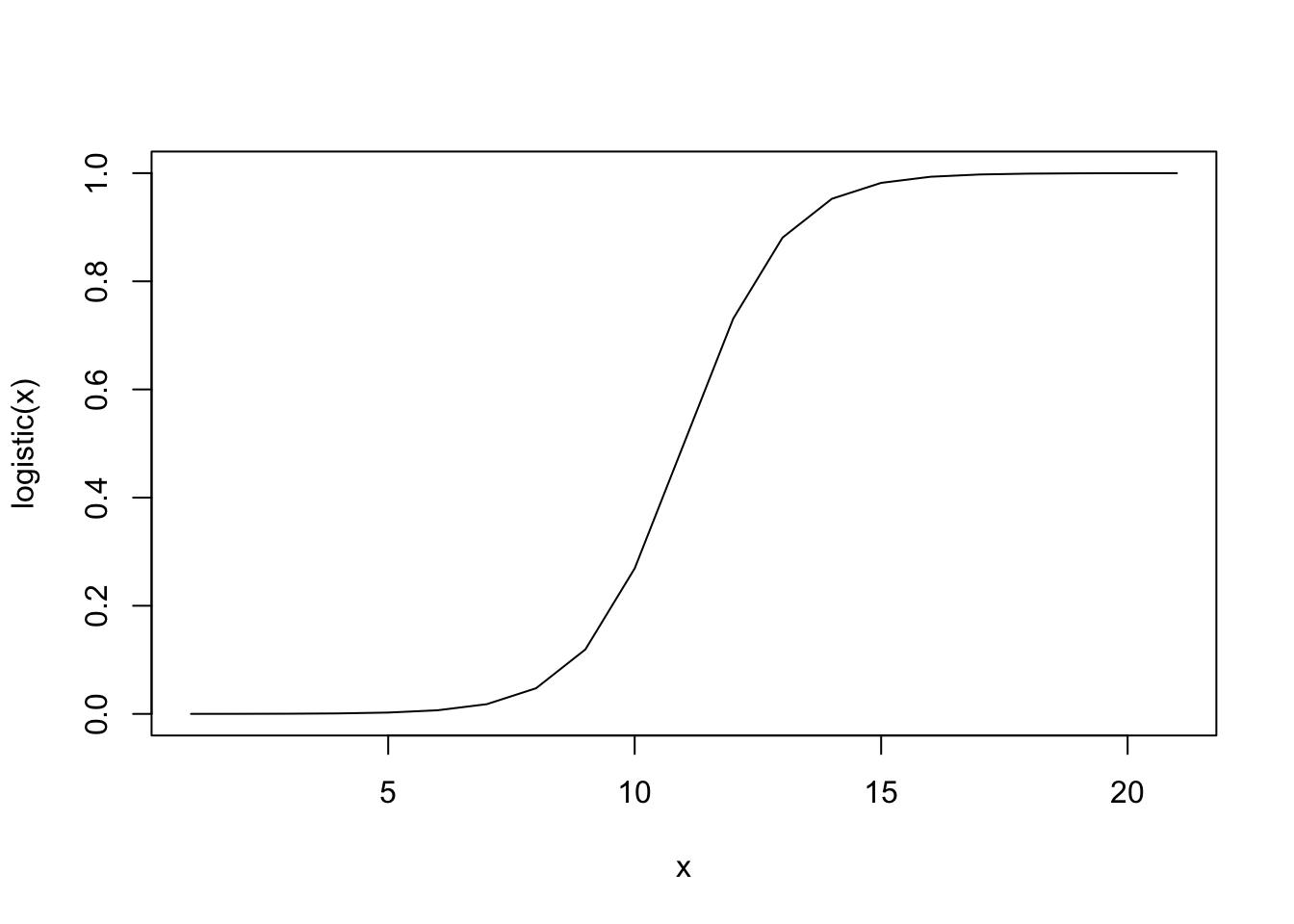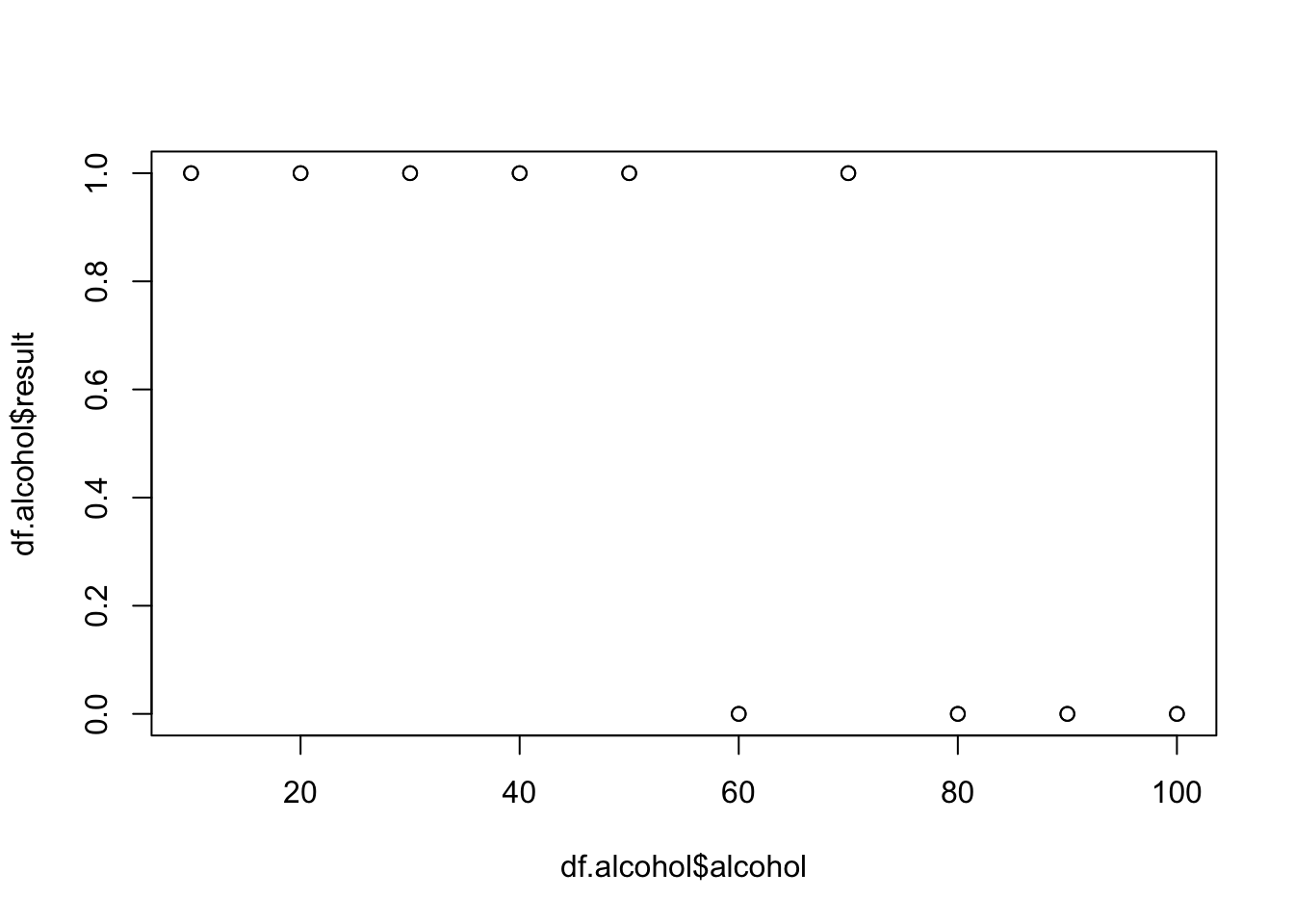# A model for almost every occasion

Statistical models describe data and relationships within data in a simple way. Different models are required for different types of data. Last workshop we looked at the bivariate regression. But what do we do to model data where:

• there is more than one predictor?

To deal with these types of data we need to go beyond the bivariate regression.

Deciding which model to use is challenging. Thankfully, statistical textbooks often have decision trees to guide you. I rather like the book Discovering Statistics using R and I have uploaded the decision tree at https://files.warwick.ac.uk/jamestripp/files/DecisionTree-DiscoveringStatisticsUsingR.pdf for your viewing pleasure.

Note: This decision tree is from a textbook written by a psychologist and so you can replace ANOVA with linear regression in most cases.

In this document, we will use a logistic regression. Following the above decision tree it tells us that logistic regressions are suitable for data with a categorical outcome, and one or more predictors which are either categorical or continious (e.g., predicting yes/no responses using likert scale responses).

## Logistic regression

Logistic regression is used when the outcome is categorical - such as a yes or no answer - and the predictor is continious - such as age or a likert scale response.

In a logistic regression, the linear regression predictions are passed through a logit function. That sounds tricky. In reality a logit function scales value between 0 and 1 in a sinusoidal way. The function is called a link function - it links the regression predictions to the data.

To remind ourselves, this is the linear regression equation with one predictor and outcome:

$outcome_i = \alpha + \beta \cdot predictor_i + \epsilon$

For a logistic regression this becomes,

$P(outcome_i) = \frac{1}{1 + \exp^{-(\beta \cdot predictor_i + \alpha)}} + \epsilon$

where the probablity of choosing the outcome (1) is the result the regression put through a logistic function.

So, what is a logistic function. Simply put, it takes in values and gives us results which between 0 and 1 in an S shape.

logistic <- function(x){
1/(1 + exp(-x))
}
plot(logistic(-10:10), type = 'l', xlab = 'x', ylab = 'logistic(x)')I like to think of it as a quick fix someone put together - possibly in a pub - to make a regression work with those darned binary (0 and 1) variables.

# Our example

We are going to fit and explore a basic logistic regression model. We need data for this.

Let’s return to our ‘drinking approach’ from the linear regression workshop. Last time we asked our fictional people to play a computer game. I think we were too kind to them last time. Instead, we will ask them to solve a complex maths question. They can either fail or succeed.

1. We recruit 20 students.
2. Each student is given a different amount of alcohol mixed with water.
3. Participant try to complete a complex maths question. They either succeed (1) or fail(0).

Let’s clear our R enviroment.

rm(list=ls())

and define our hypothetical data.

df.alcohol <- data.frame(alcohol = c(10, 10,  20, 20, 30, 30, 40, 40, 50, 50, 60, 60, 70, 70, 80, 80, 90, 90, 100, 100),
result = c(1, 1, 1, 1, 1, 1, 1, 1, 1, 1, 0, 0, 1, 1, 0, 0, 0, 0, 0, 0))

# Analysis

## Looking at the data

What do we have? We can print out the data

df.alcohol

but it probably makes more sense to tidy it up. Those 0s and 1s should be fail or succeed. Factor allows us to deal with this.

df.alcohol$recode <- factor(df.alcohol$result, labels = c('Fail', 'Succeed'))

We should double check that works

df.alcohol

and plot the data if we have lots of data.

plot(df.alcohol$alcohol, df.alcohol$result)or to look at it another way,

table(df.alcohol$alcohol, df.alcohol$recode)
##
##       Fail Succeed
##   10     0       2
##   20     0       2
##   30     0       2
##   40     0       2
##   50     0       2
##   60     2       0
##   70     0       2
##   80     2       0
##   90     2       0
##   100    2       0

What do you think is happening? Does alcohol free the mind and enable us to overcome the complexity of math? Or is it an inhibiter to all things numeric?

## Modeling

A logistic regression is a generalized linear model. We have taken our basic linear model and generalized it by putting the predictions through a logistic function. Unsuprisingly, the function we use for a logistic model is glm (generalized linear model). We can fit the model like so:

m1 <- glm(result~alcohol, data = df.alcohol, family = 'binomial')

You may - quite resonably - be wondering what the family argument does. The logistic function gives us values between 0 and 1. The binomial probability distribution allows us find the probablity of these value given the data. In essance, it is useful for fitting the model. Exactly why goes beyond this workshop.

### Interpreting the results

Does alcohol help bring out the genius in us all?

summary(m1)
##
## Call:
## glm(formula = result ~ alcohol, family = "binomial", data = df.alcohol)
##
## Deviance Residuals:
##      Min        1Q    Median        3Q       Max
## -1.46407  -0.27804   0.05822   0.27672   1.45970
##
## Coefficients:
##             Estimate Std. Error z value Pr(>|z|)
## (Intercept)  8.42521    3.95534   2.130   0.0332 *
## alcohol     -0.12954    0.05975  -2.168   0.0301 *
## ---
## Signif. codes:  0 '***' 0.001 '**' 0.01 '*' 0.05 '.' 0.1 ' ' 1
##
## (Dispersion parameter for binomial family taken to be 1)
##
##     Null deviance: 26.920  on 19  degrees of freedom
## Residual deviance: 10.028  on 18  degrees of freedom
## AIC: 14.028
##
## Number of Fisher Scoring iterations: 6

1. The coefficients are in units before the logistic function is applied. You can convert the coefficient to probability as follows.
logit2prob <- function(logit){
odds <- exp(logit)
prob <- odds / (1 + odds)
return(prob)
}

logit2prob(coef(m1))
## (Intercept)     alcohol
##   0.9997808   0.4676593

Though most people do not do this and it is tricky to interpret.

1. The AIC is a penalised measure of model fit. The details go beyond this session. Essentailly the log likelihood of the data given the model is added the the number of free parameters. You probably do not need to know this at this point. Feel free to forget the last sentence (or google it for more fun!).

Note: You are very welcome to skip the next few paragraphs and go onto plots.

We can calculate something like R for logistic regression. The statistic is called McFadden’s R squared. We can use code shown here and for those geeks like me who like lists, there is a list of the commonly encountered R squared values here.

We take the ratio of a ‘measure of the model fit’ (the log likelihood; not exactly a measure of fit but close) for our model with and without alcohol as a predictor. If this ratio is 1 then the models fit the data equally well, and 1 - this ratio = 0. Better fits produce smaller log likelihoods. A better fitting model (vs the null model) gives use values approaching 1 because the value of the ratio decreases.

mod <- glm(result~alcohol, data = df.alcohol, family = binomial)
nullmod <- glm(result~1, data = df.alcohol, family = binomial)
1-logLik(mod)/logLik(nullmod)
## 'log Lik.' 0.6275107 (df=2)

### Checking our intuition

Above we talked about the equation for a logistic regression. Putting in our variable names it should be,

$P(succeed_i) = \frac{1}{1 + \exp^{-(\beta \cdot alcohol_i + \alpha)}} + \epsilon$

So we will put our money where our mouth is, so to speak. The predictions of the model are

m1$fitted.values ## 1 2 3 4 5 6 ## 0.99919974 0.99919974 0.99708316 0.99708316 0.98942777 0.98942777 ## 7 8 9 10 11 12 ## 0.96243735 0.96243735 0.87523077 0.87523077 0.65759268 0.65759268 ## 13 14 15 16 17 18 ## 0.34460204 0.34460204 0.12583590 0.12583590 0.03791607 0.03791607 ## 19 20 ## 0.01067452 0.01067452 and putting these into our logistic regression equations coef(m1) ## (Intercept) alcohol ## 8.4252071 -0.1295437 df.alcohol$pred <- 1/(1+exp(-((-0.1295437*df.alcohol$alcohol) + 8.4252071))) Success. We have the correct logistic regression equation. ### Plots! Enough nerding out, let’s create some nice plots of our data, model and confidence intervals. Like the standard regression, we can generate our predicted data and confidence interval. df.new <- data.frame(alcohol = c(10, 20, 30, 40, 50, 60, 70, 80, 90, 100)) preds <- predict(m1, df.new, type="response", se.fit=TRUE) #For the plot, I want the predicted probabilities +/- 1.96 standard errors (that’s the 95% confidence interval; use qnorm(0.975) if 1.96 is not precise enough). I extract and calculate the values for each line separately to better understand the code. - taken from https://druedin.com/2016/01/16/predicted-probabilities-in-r/ predf <- preds$fit # predicted
lower <- preds$fit - (1.96*preds$se.fit) # lower bounds
upper <- preds$fit + (1.96*preds$se.fit) # upper bounds

plot(seq(from=10, to = 100, by = 10), preds\$fit, type="l", ylab="Predicted Probability to succeed on maths question", xlab="Alcohol(ml)", bty="n")
lines(seq(from=10, to = 100, by = 10), lower, lty=2, col = 'red')
lines(seq(from=10, to = 100, by = 10), upper, lty=2, col = 'red')
points(df.alcohol, col = 'blue')